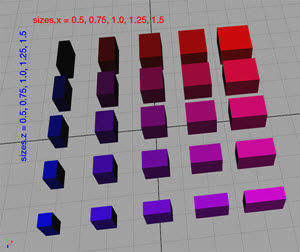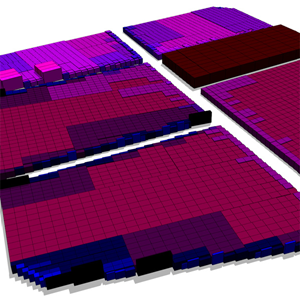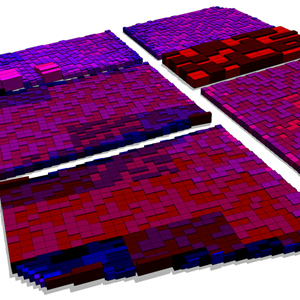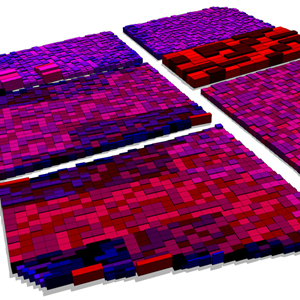# assetApproxSize function

### Syntax

• string assetApproxSize(searchQuery, axisSize, n)

### Parameters

1. searchQuerystring
Search query to apply on list of all files in the workspace. See fileSearch / filesSearch for details about the syntax.
2. axisSizestring
{ "x" | "y" | "z" | "xy" | "yz" | "xyz" }—Axes for the current scope.
3. nfloat
Number (integer >= 1) of possible returned result strings (1 returned randomly out of n possibilities).

### Returns

Asset with one of the best n size fits (according to axisSize).

### Description

The assetApproxSize function returns one of the n best size fitting assets, from the files specified in searchQuery, according to the specified axisSize string.

##### Note:

assetApproxSize(searchQuery, axesSize, 1) == assetBestSize(searchQuery, axesSize)

### Examples

#### Inserting assets based on their (physical) size

 The goal is to insert assets from a pool, depending on their (physical) size. The pool of assets is seen in the following image. Colors are (only) used to visually emphasize the size ratio.##### Note:
• Note the geometry variations in the next 3 images by using only the n parameter.
• Note that small parts get blue assets while large parts get red assets.

#### n = 1: "best fit"

 ``````Lot --> s('0.9,'0.9,'0.9) center(xz) RecursiveSplit RecursiveSplit --> case scope.sx >= 1.5 && scope.sz >= 1.5: split(x) { ~scope.sx/3 : split(z) { ~scope.sz/3 : RecursiveSplit }* }* else: innerRectangle(scope) { shape : Insert } Insert --> alignScopeToAxes(y) i(assetApproxSize( "/myProject/assets/cube_*.obj", "xz", 1))``````#### n=2: "choose randomly one of the best two fits"

 ``````Insert --> alignScopeToAxes(y) i(assetApproxSize( "/myProject/assets/cube_*.obj", "xz", 2))``````#### n=3: "choose randomly one of the best three fits"

 ``````Insert --> alignScopeToAxes(y) i(assetApproxSize( "/myProject/assets/cube_*.obj", "xz", 3))``````